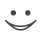Zu dieser Karteikarte gibt es einen kompletten Satz an Karteikarten. Kostenlos!

230
10.A variable x starts at zero and follows the generalized Wiener process
dx = a dt + b dz
where time is measured in years. During the first two years a=3 and b=4. During the following three years a=6 and b=3. What the standard deviation  of the value of the variable at the end of 5 years
A.6.2
B.6.7
C.7.2
D.7.7